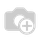• Pricing

# How set product sale price based on cost tiers.

Good day everyone,

Any idea how to implement the following logic in code or configuration  to set the product sale priced based on cost like this:

if price cost between 0 and 10 then set sale price as cost multiplied 4 times

if price cost between 11 and 50 then set sale price as cost multiplied 2 times

if price cost above 50 then set sale price as cost multiplied 1 time

I tried with standard pricing advanced formula without any luck so far.

Thanks in advance